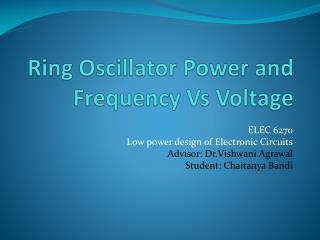DownloadDownload PresentationRing Oscillator Power and Frequency Vs Voltage

# Ring Oscillator Power and Frequency Vs Voltage

Télécharger la présentation## Ring Oscillator Power and Frequency Vs Voltage

- - - - - - - - - - - - - - - - - - - - - - - - - - - E N D - - - - - - - - - - - - - - - - - - - - - - - - - - -
##### Presentation Transcript

1. Ring Oscillator Power and Frequency Vs Voltage ELEC 6270 Low power design of Electronic Circuits Advisor: Dr.Vishwani Agrawal Student: Chaitanya Bandi

2. Index • Ring Oscillator Operation • Tools Used • Schematic • Power and Frequency Versus Voltage (Expected and Observed) for different values of N (where N is the number of gates). • References

3. Ring Oscillator • A ring oscillator is a device composed of an odd number of NOT gates whose output oscillates between two voltage levels, representing 1 and 0. • The NOT gates, or inverters, are attached in a chain; the output of the last inverter is fed back into the first

4. Tools Used • Design Architect: To create and Edit the Schematic • Eldo: To find the Frequency of oscillations and Average Power consumed

5. Schematic from Design Architect Ringin: input pin to initialize the oscillations Ringout: output pin from at which oscillations are observed

6. Simulation results of the Ring oscillator from ELDO for N=11 Vdd= 5 v Vdd= 4v

7. Vdd = 3 v Vdd=2v

8. Vdd= 1 v Vdd = .9 v

9. Vdd= .85v Vdd= .815

10. Rise time & Fall Time Vs Voltage N=11 The rise times and fall times of the oscillator increases with a decrease in supply voltage which indicates that the delay of the gates increases with a decrease in the supply voltage.

11. For N=11, Power & Freq Vs Voltage

12. For N=19, Power & Freq Vs Voltage

13. For N=5, Power & Freq Vs Voltage

14. N=19,11,5 Power Vs Voltage X-Axis: Voltage(Volts) Y-Axis: Power(uW)

15. N=19,11,5 Frequency Vs Voltage X-Axis: Voltage(Volts) Y-Axis: Frequency(MHz)

16. Power Vs number of gates(N) X-axis: Number of gates Y-axis: Average Power As the number of gates increases the Average power increases proportionally.

17. Expected Power and Frequency • Power α f* (Vdd) ^2 ; where f is the observed frequency • 2) Delay of Each gate α (Vdd)/(Vdd-Vth) • Vth = 0.54v for tsmc035 technology

18. Average power & Expected Power N=11

19. Average power & Expected Power N=19

20. Average power & Expected Power N=5

21. Observed & Expected Frequency N=11

22. Observed & Expected Frequency N=19

23. Observed & Expected Frequency N=5

24. Observations • If the Vdd is changed from 5v to 0.85V there is a power reduction of about 99.9%, but the frequency of oscillations is decreased by 76% . • The Frequency of oscillations decreases as the number of gates in the oscillator increases. • The Power consumption increases in proportion to the number of gates in the oscillator. • The Observed frequencies are lesser than the expected frequencies due to large delays in the gates at low operating voltages.

25. References: • Fall 2007: ELEC6270 Low Power Design Electronics Class Slides from Dr. Agrawal • Fall 2007: ELEC6250 Computer Aided Design of Digital Logic Circuits Lectures 4,23 from Dr. Nelson’s Class slides. • http://www.iue.tuwien.ac.at/phd/martins/node46.html